# Class 8 RD Sharma Solutions – Chapter 17 Understanding Shapes Special Types Of Quadrilaterals – Exercise 17.1 | Set 2

• Last Updated : 16 Apr, 2021

### Question 16. The perimeter of a parallelogram is 150 cm. One of its sides is greater than the other by 25 cm. Find the length of the sides of the parallelogram.

Solution:

Given that,

Attention reader! All those who say programming isn't for kids, just haven't met the right mentors yet. Join the  Demo Class for First Step to Coding Coursespecifically designed for students of class 8 to 12.

The students will get to learn more about the world of programming in these free classes which will definitely help them in making a wise career choice in the future.

Perimeter of the parallelogram = 150 cm

Let us assume that one of the sides as = ‘x’ cm

and other side as = (x + 25) cm

As we know that opposite sides of a parallelogram are parallel and equal.

Therefore, Perimeter = Sum of all sides

x + x + 25 + x + x + 25 = 150

4x + 50 = 150

4x = 150 – 50

x = 100/4 = 25

Hence, Sides of the parallelogram are (x) = 25 cm and (x+25) = 50 cm.

### Question 17. The shorter side of a parallelogram is 4.8 cm and the longer side is half as much again as the shorter side. Find the perimeter of the parallelogram.

Solution:

Given that,

Shorter side of the parallelogram = 4.8 cm

and longer side of the parallelogram = 4.8 + 4.8/2 = 4.8 + 2.4 = 7.2cm

As we know that opposite sides of a parallelogram are parallel and equal.

Therefore, Perimeter = Sum of all sides

Perimeter of the parallelogram = 4.8 + 7.2 + 4.8 + 7.2 = 24cm

Hence, Perimeter of the parallelogram is 24 cm.

### Question 18. Two adjacent angles of a parallelogram are (3x-4)o and (3x+10)°. Find the angles of the parallelogram.

Solution:

As we know that adjacent angles of a parallelogram are equal.

Therefore, (3x – 4)° + (3x + 10)° = 180°

3x° + 3xo – 4 + 10 = 180°

6x = 180° – 6°

x = 174°/6 = 29°

(3x – 4)° = 3×29 – 4 = 83°

(3x + 10)° = 3×29 + 10 = 97°

As we know that Sum of adjacent angles = 180°

Hence, each angle is 83°, 97°, 83°, 97°.

### Question 19. In a parallelogram ABCD, the diagonals bisect each other at O. If ∠ABC =30°, ∠BDC= 10° and ∠CAB =70°. Find:

∠DAB, ∠ADC, ∠BCD, ∠AOD, ∠DOC, ∠BOC, ∠AOB, ∠ACD, ∠CAB, ∠ADB, ∠ACB, ∠DBC, and ∠DBA.

Solution: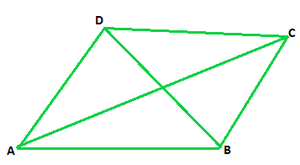Given that,

∠ABC = 30°,

∠ABC = ∠ADC = 30° (We know that measure of opposite angles are equal in a parallelogram)

∠BDC = 10°

∠CAB =70°

∠BDA = ∠ADB = ∠ADC – ∠BDC = 30° – 10° = 20° (From figure we concluded)

∠DAB = 180° – 30° = 150°

∠ADB = ∠DBC = 20° (alternate angles)

∠BCD = ∠DAB = 150° (we know, opposite angles are equal in a parallelogram)

∠DBA = ∠BDC = 10° (we know, Alternate interior angles are equal)

In ΔABC ∠CAB + ∠ABC + ∠BCA = 180° (since, sum of all angles of a triangle is 180°)

70° + 30° + ∠BCA = 180°

∠BCA = 180° – 100° = 80°

∠DAB = ∠DAC + ∠CAB = 70° + 80° = 150°

∠BCD = 150° (opposite angle of the parallelogram)

∠DCA = ∠CAB = 70°

In ΔDOC ∠BDC + ∠ACD + ∠DOC = 180° (since, sum of all angles of a triangle is 180°)

10° + 70° + ∠DOC = 180°

∠DOC = 180°- 80°

∠DOC = 100°

Therefore, ∠DOC = ∠AOB = 100° (Vertically opposite angles are equal)

∠DOC + ∠AOD = 180° [Linear pair]

100° + ∠AOD = 180°

∠AOD = 180°- 100°

∠AOD = 80°

Therefore, ∠AOD = ∠BOC = 80° (Vertically opposite angles are equal)

∠CAB = 70o

∠ABC + ∠BCD = 180° (In a parallelogram sum of adjacent angles is 180°)

30° + ∠ACB + ∠ACD = 180°

30° + ∠ACB + 70° = 180°

∠ACB = 180° – 100°

∠ACB = 80°

Hence ∠DAB = 150o, ∠ADC = 30°, ∠BCD = 150°, ∠AOD = 80°, ∠DOC = 100°, ∠BOC = 80°, ∠AOB = 100°, ∠ACD = 70°, ∠CAB = 70°, ∠ADB = 20°, ∠ACB = 80°, ∠DBC = 20°, and ∠DBA = 10°.

### Question 20. Find the angles marked with a question mark shown in Figure.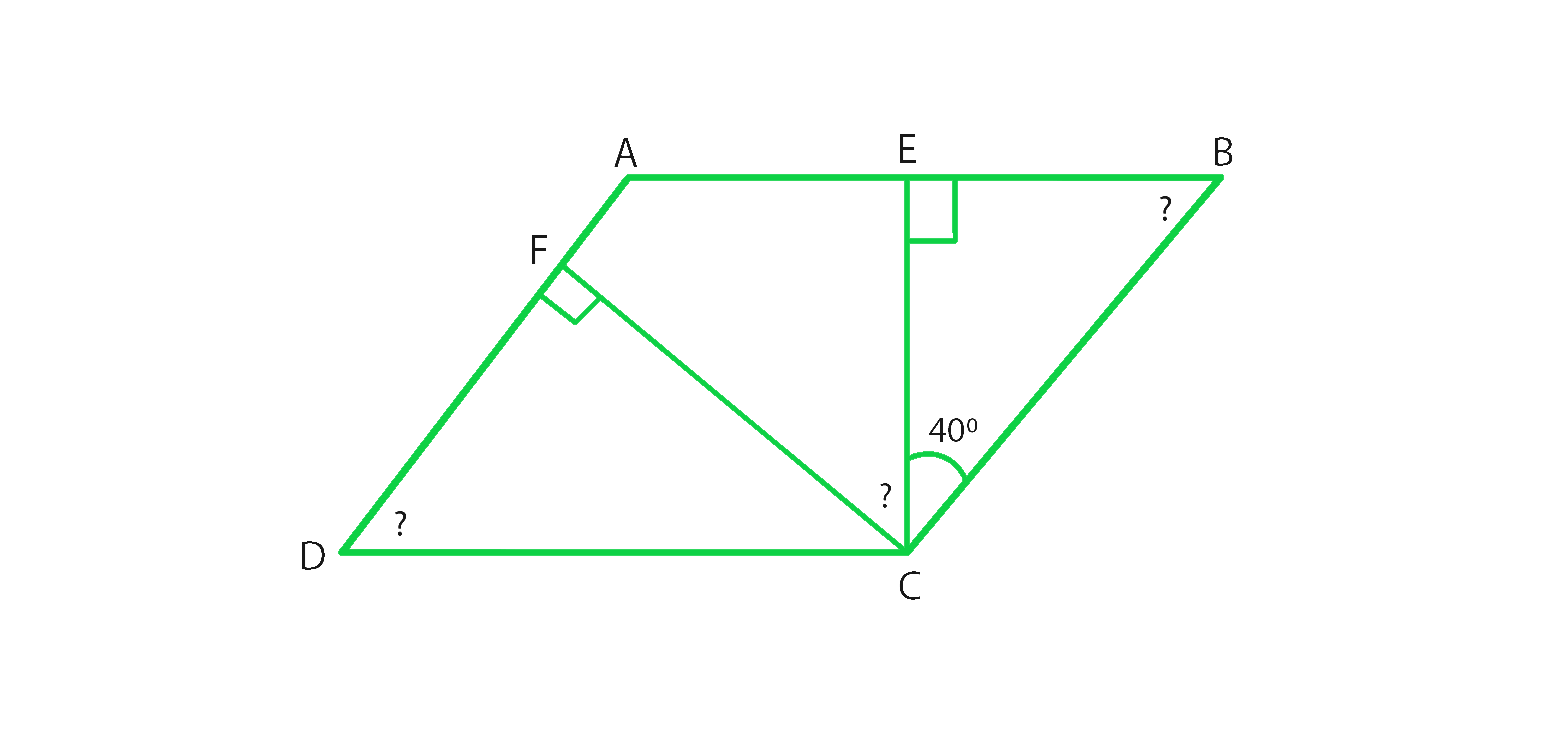Solution:

In ΔBEC ∠BEC + ∠ECB +∠CBE = 180° (Sum of angles of a triangle is 180°)

90° + 40° + ∠CBE = 180°

∠CBE = 180°-130°

∠CBE = 50°

∠CBE = ∠ADC = 50° (Opposite angles of a parallelogram are equal)

∠B = ∠D = 50° (opposite angles of a parallelogram are equal)

∠A + ∠B = 180° (Sum of adjacent angles of a triangle is 180°)

∠A + 50° = 180°

∠A = 180°-50°

therefore, ∠A = 130°

In ΔDFC ∠DFC + ∠FCD +∠CDF = 180° (Sum of angles of a triangle is 180°)

90° + ∠FCD + 50° = 180°

∠FCD = 180°-140°

∠FCD = 40°

∠A = ∠C = 130° (Opposite angles of a parallelogram are equal)

∠C = ∠FCE +∠BCE + ∠FCD

∠FCD + 40° + 40° = 130°

∠FCD = 130° – 80°

∠FCD = 50°

Hence ∠EBC = 50°, ∠ADC = 50° and ∠FCD = 50°.

### Question 21. The angle between the altitudes of a parallelogram, through the same vertex of an obtuse angle of the parallelogram, is 60°. Find the angles of the parallelogram.

Solution: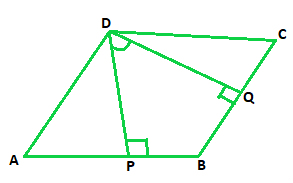Let us consider parallelogram ABCD, where DP⊥ AB and DQ ⊥ BC.

Given that ∠PDQ = 60°

In quadrilateral DPBQ ∠PDQ + ∠DPB + ∠B + ∠BQD = 360° (Sum of all the angles of a Quadrilateral is 360°)

60° + 90° + ∠B + 90° = 360°

∠B = 360° – 240°

∠B = 120°

∠B = ∠D = 120° (Opposite angles of parallelogram are equal)

∠B + ∠C = 180° (Sum of adjacent interior angles in a parallelogram is 180°)

120° + ∠C = 180°

∠C = 180° – 120° = 60°

∠A = ∠C = 60° (Opposite angles of parallelogram are equal)

Hence, Angles of a parallelogram are 60°, 120°, 60°, 120°

### Question 22. In Figure, ABCD and AEFG are parallelograms. If ∠C =55°, what is the measure of ∠F?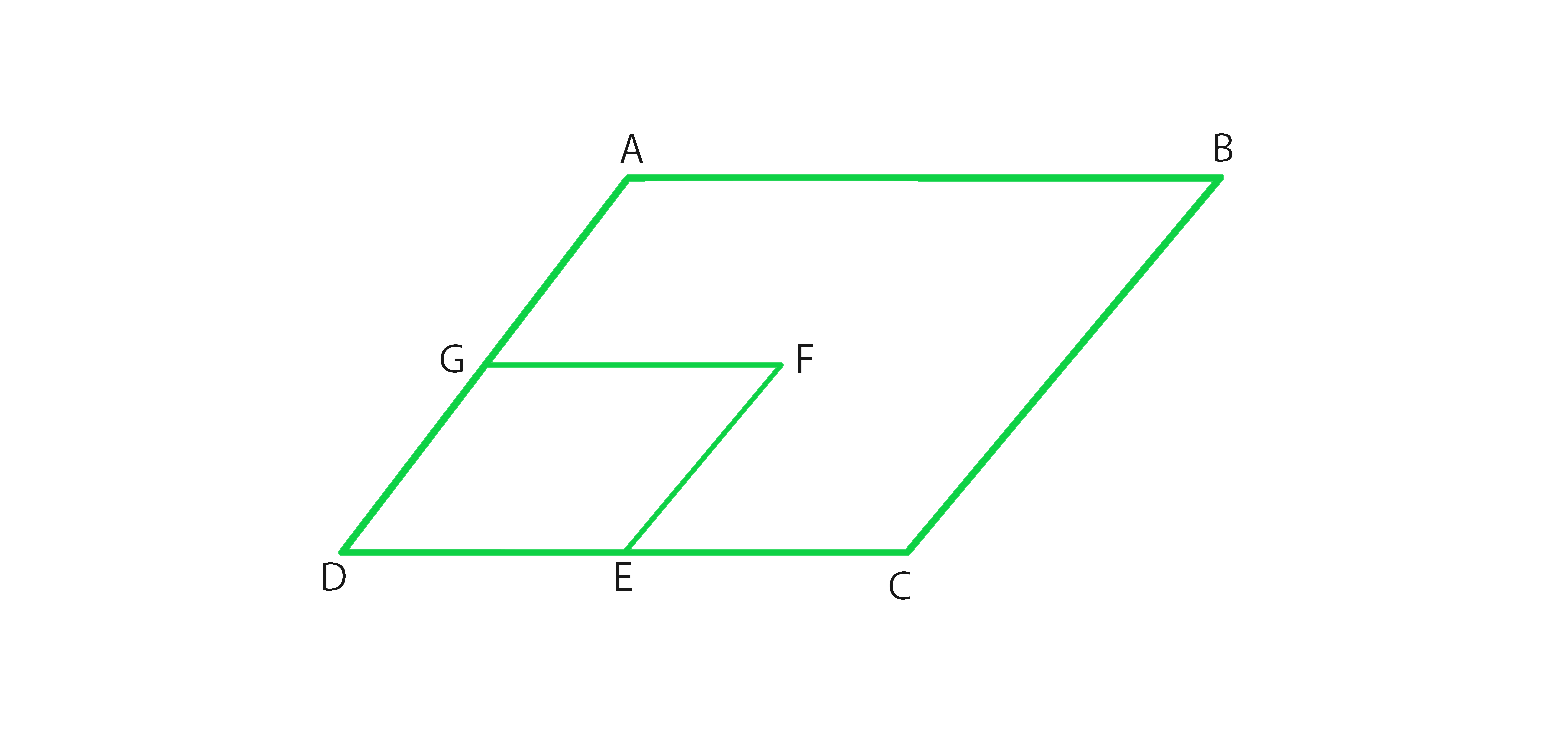Solution:

From figure, we conclude that,

In parallelogram ABCD ∠C = ∠A = 55° (In a parallelogram opposite angles are equal in a parallelogram)

In parallelogram AEFG ∠A = ∠F = 55° (In a parallelogram opposite angles are equal in a parallelogram)

Hence ∠F = 55°

### Question 23. In Figure, BDEF and DCEF are each a parallelogram. Is it true that BD = DC? Why or why not?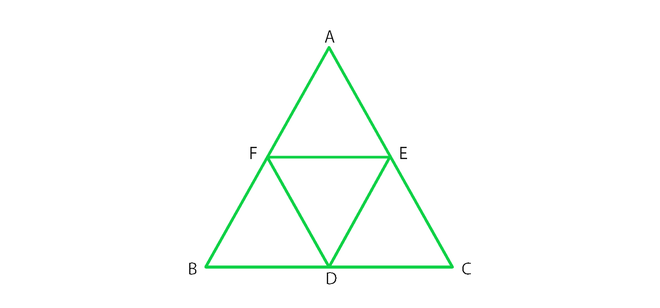Solution:

From figure, we conclude that,

In parallelogram BDEF BD = EF (In a parallelogram opposite sides are equal)

In parallelogram DCEF DC = EF (In a parallelogram opposite sides are equal)

Since, BD = EF = DC

Therefore, BD = DC

### Question 24. In Figure, suppose it is known that DE = DF. Then, is ΔABC isosceles? Why or why not?Solution:

From figure we conclude that,

In parallelogram BDEF BD = EF and BF = DE (opposite sides are equal in a parallelogram)

In parallelogram DCEF DC = EF and DF = CE (opposite sides are equal in a parallelogram)

In parallelogram AFDE AF = DE and DF = AE (opposite sides are equal in a parallelogram)

therefore, DE = AF = BF

similarly, DF = CE = AE

Given that, DE = DF

Since, DF = DF

AF + BF = CE + AE

AB = AC

Hence, ΔABC is an isosceles triangle.

### Question 25. Diagonals of parallelogram ABCD intersect at O as shown in Figure. XY contains O, and X, Y are points on opposite sides of the parallelogram. Give reasons for each of the following:

(i) OB = OD

(ii) ∠OBY = ∠ODX

(iii) ∠BOY = ∠DOX

(iv) ΔBOY = ΔDOX

Now, state if XY is bisected at O.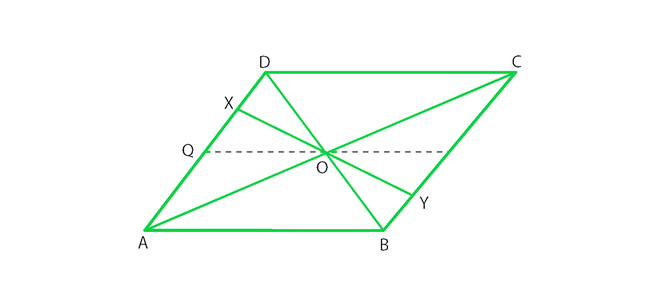Solution:

(i) OB = OD

OB = OD. Since diagonals bisect each other in a parallelogram.

(ii) ∠OBY =∠ODX

∠OBY =∠ODX. Since alternate interior angles are equal in a parallelogram.

(iii) ∠BOY= ∠DOX

∠BOY= ∠DOX. Since vertical opposite angles are equal in a parallelogram.

(iv) ΔBOY ≅ ΔDOX

ΔBOY and ΔDOX. Since OB = OD, where diagonals bisect each other in a parallelogram.

∠OBY =∠ODX (Alternate interior angles are equal)

∠BOY= ∠DOX (Vertically opposite angles are equal)

ΔBOY ≅ΔDOX (by ASA congruence rule)

OX = OY (Corresponding parts of congruent triangles)

Hence XY is bisected at O.

### Question 26. In Fig., ABCD is a parallelogram, CE bisects ∠C and AF bisects ∠A. In each of the following, if the statement is true, give a reason for the same:

(i) ∠A = ∠C

(ii) ∠FAB = ½ ∠A

(iii) ∠DCE = ½ ∠C

(iv) ∠CEB = ∠FAB

(v) CE ∥ AF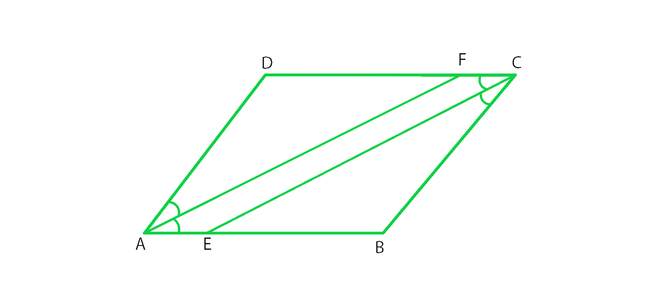Solution:

(i) ∠A = ∠C

True, Since ∠A =∠C = 55° [opposite angles are equal in a parallelogram]

(ii) ∠FAB = ½ ∠A

True, Since AF is the angle bisector of ∠A.

(iii) ∠DCE= ½ ∠C

True, Since CE is the angle bisector of angle ∠C.

(iv) ∠CEB= ∠FAB

True, Since ∠DCE = ∠FAB (opposite angles are equal in a parallelogram).

∠CEB = ∠DCE (alternate angles)

½ ∠C = ½ ∠A [AF and CE are angle bisectors]

(v) CE || AF

True, since one pair of opposite angles are equal, therefore quad. AEFC is a parallelogram.

### Question 27. Diagonals of a parallelogram ABCD intersect at O. AL and CM are drawn perpendiculars to BD such that L and M lie on BD. Is AL = CM? Why or why not?

Solution: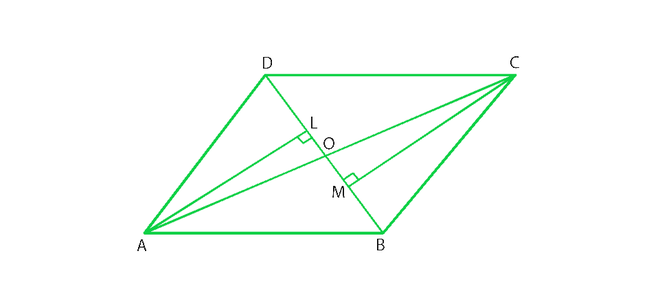Given that,

AL and CM are perpendiculars on diagonal BD.

In ΔAOL and ΔCOM,

∠AOL = ∠COM (vertically opposite angle) ———–(i)

∠ALO = ∠CMO = 90° (each right angle) ——–(ii)

By using angle sum property,

∠AOL + ∠ALO + ∠LAO = 180° ———(iii)

∠COM + ∠CMO + ∠OCM = 180° ———- (iv)

From (iii) and (iv)

∠AOL + ∠ALO + ∠LAO = ∠COM + ∠CMO + ∠OCM

∠LAO = ∠OCM (from (i) and (ii))

In ΔAOL and ΔCOM

∠ALO = ∠CMO (each right angle)

AO = OC (diagonals of a parallelogram bisect each other)

∠LAO = ∠OCM (proved)

therefore, ΔAOL is congruent to ΔCOM

Hence AL = CM (Corresponding parts of congruent triangles)

### Question 28. Points E and F lie on diagonals AC of a parallelogram ABCD such that AE = CF. what type of quadrilateral is BFDE?

Solution: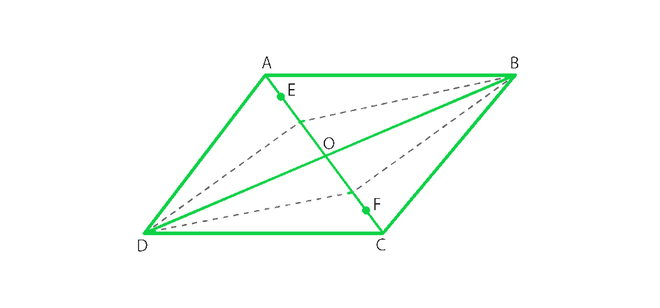From figure, we conclude that,

In parallelogram ABCD,

AO = OC ———- (i) (Diagonals of a parallelogram bisect each other)

AE = CF ———-(ii) Given

On subtracting (ii) from (i)

AO – AE = OC – CF

EO = OF ——-(iii)

In ΔDOE and ΔBOF,

EO = OF (proved)

DO = OB (Diagonals of a parallelogram bisect each other)

∠DOE = ∠BOF (vertically opposite angles are equal in a parallelogram)

By SAS congruence ΔDOE ≅ ΔBOF

therefore, DE = BF (Corresponding parts of congruent triangles)

In ΔBOE and ΔDOF,

EO = OF (proved)

DO = OB (diagonals of a parallelogram bisect each other)

∠DOF = ∠BOE (vertically opposite angles are equal in a parallelogram)

By SAS congruence ΔDOE ≅ ΔBOF

Hence, DF = BE (Corresponding parts of congruent triangles).

Hence, BFDE is a parallelogram, since one pair of opposite sides are equal and parallel.

### Question 29. In a parallelogram ABCD, AB = 10cm, AD = 6 cm. The bisector of ∠A meets DC in E, AE and BC produced meet at F. Find the length CF.

Solution: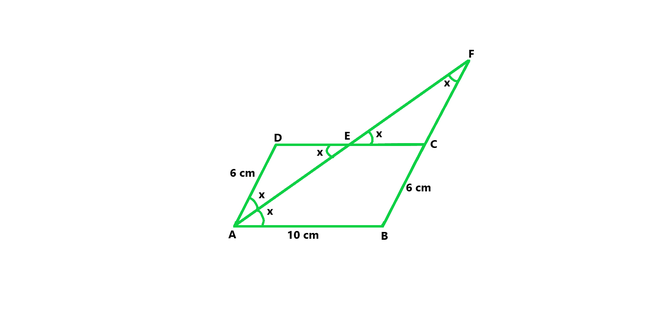From figure we concluded that,

In a parallelogram ABCD,

Given, AB = 10 cm, AD = 6 cm

CD = AB = 10 cm and AD = BC = 6 cm (In a parallelogram opposite sides are equal)

AE is the bisector of ∠DAE = ∠BAE = x

∠BAE = ∠AED = x (alternate angles are equal)

ΔADE is an isosceles triangle. Since opposite angles in ΔADE are equal.

AD = DE = 6cm (opposite sides are equal)

CD = DE + EC

EC = CD – DE = 10 – 6 = 4cm

∠DEA = ∠CEF = x (vertically opposite angle are equal)

∠EAD = ∠EFC = x (alternate angles are equal)

ΔEFC is an isosceles triangle. Since opposite angles in ΔEFC are equal.

CF = CE = 4cm (opposite side are equal to angles)

Hence CF = 4cm.

My Personal Notes arrow_drop_up